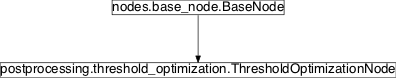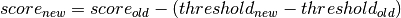# threshold_optimization¶

## Module: `missions.nodes.postprocessing.threshold_optimization`¶

Optimize classification thresholds

Inheritance diagram for `pySPACE.missions.nodes.postprocessing.threshold_optimization`:## `ThresholdOptimizationNode`¶

class `pySPACE.missions.nodes.postprocessing.threshold_optimization.``ThresholdOptimizationNode`(metric='Balanced_accuracy', class_labels=None, preserve_score=False, classifier_threshold=0.0, recalibrate=False, weight=0.5, inverse_metric=False, **kwargs)[source]

Optimize the classification threshold for a specified metric

This node changes the classification threshold (i.e. the mapping from real valued classifier prediction onto class label) by choosing a threshold that is optimal for a given metric on the training data. This may be useful in situations when a classifier tries to optimize a different metric than the one one is interested. However, it is always preferable to use a classifier that optimizes for the right target metric since this node can only correct the threshold but not the hyperplane.

If store is set to true, a graphic is stored in the persistency directory that shows the mapping of threshold onto F-Measure on training and test data.

Parameters

metric: class_labels: A string that determines the metric for which the threshold is optimized. The string must be a valid Python expression that evaluates to a float. Within this string, the quantities {TP} (true positive), {FP} (false positives), {TN} (true negatives), and {FN} (false negatives) can be used to compute the the metric. For instance, the string “({TP}+{TN})/({TP}+{TN}+{FP}+{FN})” would correspond to the accuracy. Some standard metrics (F-Measure, Accuracy) are predefined, i.e. it suffices to give the names of these metrics as parameter, the corresponding Python expression is determined automatically. For details and inspiration have a look at metric in the `BinaryClassificationDataset`. Warning If your metric is not existing, the algorithm will get zero instead and will get problems optimizing. This is due to the fact, that default values for metrics are zero. (optional, default: “Balanced_accuracy”) Determines the order of classes, i.e. the mapping of class labels onto integers. The first element of the list will be mapped onto 0, the second onto 1. (recommended, default: [‘Standard’, ‘Target’]) If True, only the class labels are changed according to the new threshold. If False, the classifier prediction score is also adjusted by adding the new threshold, i.e.(optional, default: False) Old decision threshold of the classifier. For SVMs this is zero. For bayesian classifier or after probability fits this is 0.5. (optional, default: 0.0) If the distribution in the incremental learning is expected to be significantly different from the training session, a new threshold is calculated using only the new examples and not considering the old ones. If the parameter is active, retrain is also active! (optional, default: False) Parameter for weighted metrics If you want to use it, have a look at metric and the `pySPACE.missions.nodes.sink.classification_performance_sink.PerformanceSinkNode` (optional, default: 0.5) For some metrics one has to optimize for a low value and not a high. This is done by multiplication with -1 in the formula or by setting this parameter to True, if you use some predefined metrics, which requires minimization.

Exemplary Call

```-
node : Threshold_Optimization
parameters :
metric : "-{FP} - 5*{FN}"
class_labels : ['Standard', 'Target']
```
Author: Created: Jan Hendrik Metzen (jhm@informatik.uni-bremen.de) 2010/11/25 Threshold_Optimization ThresholdOptimizationNode ThresholdOptimization PredictionVector

Class Components Summary

 `__hyperparameters` `_execute`(data) Shift the data with the new offset `_get_metric_fct`() `_inc_train`(data, class_label) Provide training data for retraining `_stop_training`([debug]) Call the optimization algorithm `_train`(data, class_label) Collect training data and class labels `balanced_accuracy`(TP, FP, TN, FN) `calculate_threshold`() Optimize the threshold for the given scores, labels and metric. `input_types` `is_supervised`() Returns whether this node requires supervised training `is_trainable`() Returns whether this node is trainable `start_retraining`() Start retraining phase of this node `store_state`(result_dir[, index]) Stores this node in the given directory result_dir
`input_types` = ['PredictionVector']
`__init__`(metric='Balanced_accuracy', class_labels=None, preserve_score=False, classifier_threshold=0.0, recalibrate=False, weight=0.5, inverse_metric=False, **kwargs)[source]
`balanced_accuracy`(TP, FP, TN, FN)[source]
`is_trainable`()[source]

Returns whether this node is trainable

`is_supervised`()[source]

Returns whether this node requires supervised training

`_train`(data, class_label)[source]

Collect training data and class labels

`_stop_training`(debug=False)[source]

Call the optimization algorithm

`calculate_threshold`()[source]

Optimize the threshold for the given scores, labels and metric.

Note

This method requires O(n) time (n being the number of training instances). There should be an asymptotically more efficient implementation that is better suited for fast incremental learning.

`__hyperparameters` = set([NoOptimizationParameter<kwargs_warning>, NoOptimizationParameter<dtype>, NoOptimizationParameter<output_dim>, NoOptimizationParameter<retrain>, NoOptimizationParameter<input_dim>, BooleanParameter<recalibrate>, BooleanParameter<preserve_score>, NoOptimizationParameter<store>])
`start_retraining`()[source]

Start retraining phase of this node

`_inc_train`(data, class_label)[source]

Provide training data for retraining

`_execute`(data)[source]

Shift the data with the new offset

`_get_metric_fct`()[source]
`store_state`(result_dir, index=None)[source]

Stores this node in the given directory result_dir